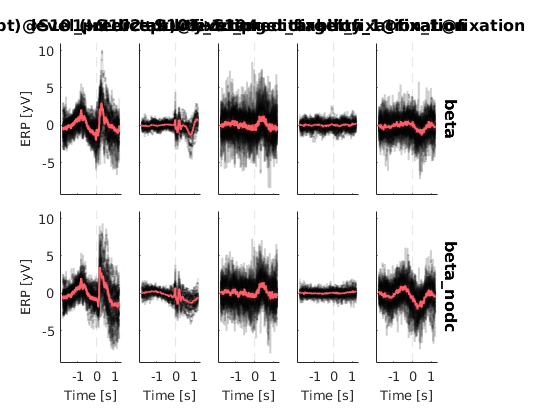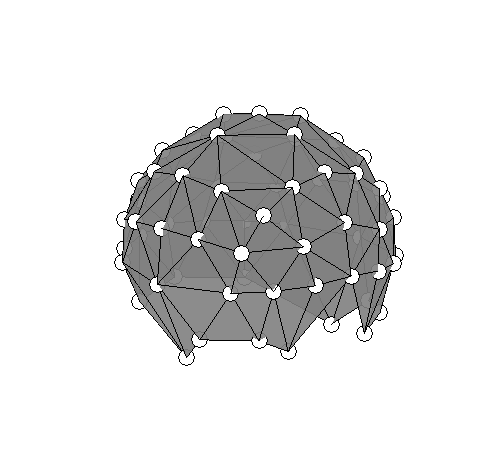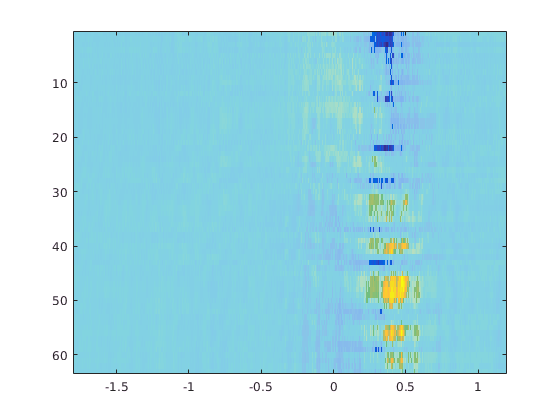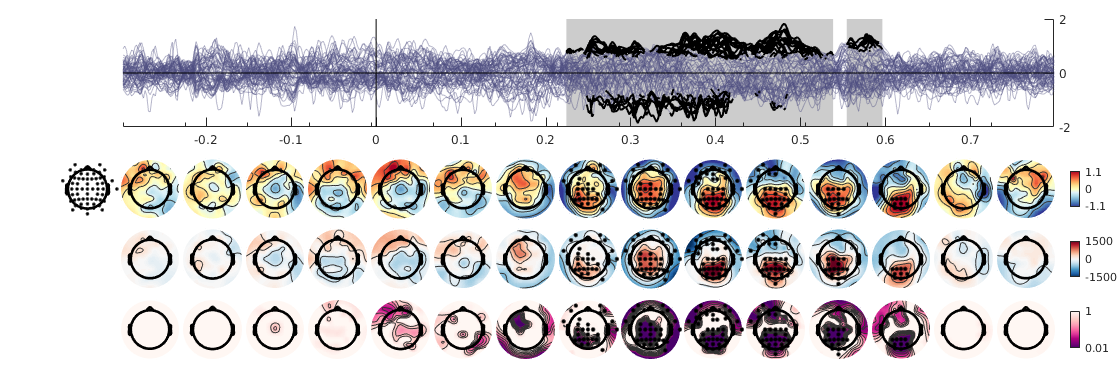The data for this tutorial are not yet publicly available sorry! I am working on a simulation for this tutorial but it might take some time!
This tutorial is in progress :)
init_unfold
Adding toolboxes and subfolders to path...
projectFolder = '/net/store/nbp/users/behinger/projects/deconvolution';
%% Load the data
d2nd = tmp.d2nd;

## Plot a single channel

%% plot deconv vs nodeconv
% This does not work with splines
cfg = [];
cfg.channel = 40; % Pz = 32
cfg.baseline = [min(d2nd.times) 0];
uf_plot2nd(d2nd,cfg);
plotting all parameters
performing baseline correction performing baseline correction
plotting all parameters
performing baseline correction performing baseline correction New X or Y of different size given, all data from first gramm cleared
ans =
gramm with properties: legend_axe_handle: [1×1 Axes] title_axe_handle: [] facet_axes_handles: [2×5 Axes] results: [1×1 struct]
Warning: A value of class "com.mathworks.mde.cmdwin.XCmdWndView" was indexed with no subscripts specified. Currently the result of this operation is the indexed value itself, but in a future release, it will be an error.
Warning: A value of class "com.mathworks.mde.cmdwin.XCmdWndView" was indexed with no subscripts specified. Currently the result of this operation is the indexed value itself, but in a future release, it will be an error.## Statistics using Threshold-Free-Cluster-Enhancement (TFCE)

% This function calculates a channel matrix that can be used for default cluster-permutation tests
% using the ept-TFCE toolbox
ept_tfce_nb = ept_ChN2(d2nd.chanlocs,1) % the 1 to plot
ept_tfce_nb =
1 3 8 13 22 0 0 0 0 2 4 12 21 28 0 0 0 0 1 3 5 6 8 0 0 0 0 2 4 6 7 12 0 0 0 0 3 5 6 8 9 0 0 0 0 3 4 5 6 7 9 10 11 0 4 6 7 11 12 0 0 0 0 1 3 5 8 9 13 14 0 0 5 6 8 9 10 14 15 16 0 6 9 10 11 16 17 18 0 0 6 7 10 11 12 18 19 20 0 2 4 7 11 12 20 21 0 0 1 8 13 14 22 23 29 0 0 8 9 13 14 15 23 24 0 0 9 14 15 16 24 0 0 0 0 9 10 15 16 17 24 25 32 0 10 16 17 18 25 0 0 0 0 10 11 17 18 19 25 26 34 0 11 18 19 20 26 0 0 0 0 11 12 19 20 21 26 27 0 0 2 12 20 21 27 28 37 0 0 1 13 22 29 64 0 0 0 0 13 14 23 24 29 30 31 0 0 14 15 16 23 24 31 32 0 0 16 17 18 25 32 33 34 0 0 18 19 20 26 27 34 35 0 0 20 21 26 27 35 36 37 0 0 2 21 28 37 43 0 0 0 0 13 22 23 29 30 38 44 64 0 23 29 30 31 38 0 0 0 0 23 24 30 31 32 38 39 0 0 16 24 25 31 32 33 39 40 47 25 32 33 34 40 0 0 0 0 18 25 26 33 34 35 40 41 49 26 27 34 35 36 41 42 0 0 27 35 36 37 42 0 0 0 0 21 27 28 36 37 42 43 52 0 29 30 31 38 39 44 45 0 0 31 32 38 39 45 46 47 0 0 32 33 34 40 47 48 49 0 0 34 35 41 42 49 50 51 0 0 35 36 37 41 42 51 52 0 0 28 37 43 52 59 0 0 0 0 29 38 44 45 53 54 64 0 0 38 39 44 45 46 54 55 0 0 39 45 46 47 55 0 0 0 0 32 39 40 46 47 48 55 56 0 40 47 48 49 56 0 0 0 0 34 40 41 48 49 50 56 57 0 41 49 50 51 57 0 0 0 0 41 42 50 51 52 57 58 0 0 37 42 43 51 52 58 59 0 0 44 53 54 60 63 64 0 0 0 44 45 53 54 55 60 0 0 0 45 46 47 54 55 56 60 61 0 47 48 49 55 56 57 61 0 0 49 50 51 56 57 58 61 62 0 51 52 57 58 59 62 0 0 0 43 52 58 59 62 63 0 0 0 53 54 55 60 61 63 0 0 0 55 56 57 60 61 62 63 0 0 57 58 59 61 62 63 0 0 0 53 59 60 61 62 63 0 0 0 22 29 44 53 64 0 0 0 0 0 0 0 0 0 0 0 0 0 0 0 0 0 0 0 0 0 0 0 0 0 0 0 0 0 0 0 0 0 0 0 0 0 0 0 0 0 0 0 0 0 0 0 0 0% Average amount of neighbours:
mean(sum(ept_tfce_nb'>0))
Warning: A value of class "com.mathworks.mde.cmdwin.XCmdWndView" was indexed with no subscripts specified. Currently the result of this operation is the indexed value itself, but in a future release, it will be an error.
Warning: A value of class "com.mathworks.mde.cmdwin.XCmdWndView" was indexed with no subscripts specified. Currently the result of this operation is the indexed value itself, but in a future release, it will be an error.
ans =
5.5938
Warning: A value of class "com.mathworks.mde.cmdwin.XCmdWndView" was indexed with no subscripts specified. Currently the result of this operation is the indexed value itself, but in a future release, it will be an error.
Warning: A value of class "com.mathworks.mde.cmdwin.XCmdWndView" was indexed with no subscripts specified. Currently the result of this operation is the indexed value itself, but in a future release, it will be an error.
Run the analysis using the ept-tfce toolbox
cfg = [];
cfg.chan = 1:63; % the other channels are eye-movement channels from the Eye-EEG toolbox
cfg.pred = 4; % predictability at a target-word
% subselect channels & predictor
data = squeeze(d2nd.beta(cfg.chan,:,cfg.pred,:));
% baseline correction
data = bsxfun(@minus,data,mean(data(:,d2nd.times<0,:),2));
% do the statistics
% this is a customized version of the original ept_tfce_diff function
[res,info] = be_ept_tfce_diff(struct('nperm',500,'neighbours',ept_tfce_nb(cfg.chan,:)),permute(data,[3 1 2]));
Calculating Actual Differences...
Calculating Permutations...
Done
Calculating P-Values and Saving...
All done!
Elapsed time is 73.376419 seconds.
Peak significance found at channel 48 at sample 1134: max-t-val(24) = 6.037, p = 0.001992
These results are now corrected for multiple comparisons of electrode x time. They are not corrected for multiple tests one might perform on the data.
% plot it
figure,
h = imagesc(d2nd.times,1:63, res.TFCE_Obs);This gives a complete picture of where effects are. But the spatial relations are not easily accessible. Topoplots are another way to display this data.
cfgPlot = [];
cfgPlot.pvalues = res.P_Values;
cfgPlot.colormap = {{'div','RdYlBu'},{'div','RdBu'},'seq'};
cfgPlot.topoalpha = 0.05; % where to put the significance dots?
cfgPlot.individualcolorscale = 'row'; % different rows have very different interpretation
cfgPlot.time = [-0.3 0.8]; % we will zoom in
plot_topobutter(cat(3,mean(data(1:63,:,:),3),res.TFCE_Obs,res.P_Values),d2nd.times,d2nd.chanlocs(1:63),cfgPlot)
Warning: When plotting pvalues in totoplot, use option 'conv' to minimize extrapolation effects Plotting Topoplots ... Elapsed time is 0.000297 seconds. Warning: When plotting pvalues in totoplot, use option 'conv' to minimize extrapolation effects
Elapsed time is 0.358530 seconds. Warning: When plotting pvalues in totoplot, use option 'conv' to minimize extrapolation effects
Elapsed time is 0.193594 seconds. Warning: When plotting pvalues in totoplot, use option 'conv' to minimize extrapolation effects
Elapsed time is 0.204268 seconds. Warning: When plotting pvalues in totoplot, use option 'conv' to minimize extrapolation effects
Elapsed time is 0.204011 seconds. Warning: When plotting pvalues in totoplot, use option 'conv' to minimize extrapolation effects
Elapsed time is 0.212305 seconds. Warning: When plotting pvalues in totoplot, use option 'conv' to minimize extrapolation effects
Elapsed time is 0.217514 seconds. Warning: When plotting pvalues in totoplot, use option 'conv' to minimize extrapolation effects
Elapsed time is 0.212385 seconds. Warning: When plotting pvalues in totoplot, use option 'conv' to minimize extrapolation effects
Elapsed time is 0.247328 seconds. Warning: When plotting pvalues in totoplot, use option 'conv' to minimize extrapolation effects
Elapsed time is 0.216414 seconds. Warning: When plotting pvalues in totoplot, use option 'conv' to minimize extrapolation effects
Elapsed time is 0.217188 seconds. Warning: When plotting pvalues in totoplot, use option 'conv' to minimize extrapolation effects
Elapsed time is 0.227991 seconds. Warning: When plotting pvalues in totoplot, use option 'conv' to minimize extrapolation effects
Elapsed time is 0.232560 seconds. Warning: When plotting pvalues in totoplot, use option 'conv' to minimize extrapolation effects
Elapsed time is 0.241134 seconds. Warning: When plotting pvalues in totoplot, use option 'conv' to minimize extrapolation effects
Elapsed time is 0.236643 seconds. Warning: When plotting pvalues in totoplot, use option 'conv' to minimize extrapolation effects
1.02s for row 1/3 Plotting Topoplots ... Elapsed time is 0.000017 seconds. Warning: When plotting pvalues in totoplot, use option 'conv' to minimize extrapolation effects
Elapsed time is 0.270511 seconds. Warning: When plotting pvalues in totoplot, use option 'conv' to minimize extrapolation effects
Elapsed time is 0.273747 seconds. Warning: When plotting pvalues in totoplot, use option 'conv' to minimize extrapolation effects
Elapsed time is 0.286562 seconds. Warning: When plotting pvalues in totoplot, use option 'conv' to minimize extrapolation effects
Elapsed time is 0.295593 seconds. Warning: When plotting pvalues in totoplot, use option 'conv' to minimize extrapolation effects
Elapsed time is 0.293765 seconds. Warning: When plotting pvalues in totoplot, use option 'conv' to minimize extrapolation effects
Elapsed time is 0.302983 seconds. Warning: When plotting pvalues in totoplot, use option 'conv' to minimize extrapolation effects
Elapsed time is 0.304064 seconds. Warning: When plotting pvalues in totoplot, use option 'conv' to minimize extrapolation effects
Elapsed time is 0.327282 seconds. Warning: When plotting pvalues in totoplot, use option 'conv' to minimize extrapolation effects
Elapsed time is 0.345243 seconds. Warning: When plotting pvalues in totoplot, use option 'conv' to minimize extrapolation effects
Elapsed time is 0.340354 seconds. Warning: When plotting pvalues in totoplot, use option 'conv' to minimize extrapolation effects
Elapsed time is 0.351449 seconds. Warning: When plotting pvalues in totoplot, use option 'conv' to minimize extrapolation effects
Elapsed time is 0.351757 seconds. Warning: When plotting pvalues in totoplot, use option 'conv' to minimize extrapolation effects
Elapsed time is 0.363332 seconds. Warning: When plotting pvalues in totoplot, use option 'conv' to minimize extrapolation effects
Elapsed time is 0.358539 seconds. Warning: When plotting pvalues in totoplot, use option 'conv' to minimize extrapolation effects
1.14s for row 2/3 Plotting Topoplots ... Elapsed time is 0.000019 seconds. Warning: When plotting pvalues in totoplot, use option 'conv' to minimize extrapolation effects
Elapsed time is 0.358107 seconds. Warning: When plotting pvalues in totoplot, use option 'conv' to minimize extrapolation effects
Elapsed time is 0.407306 seconds. Warning: When plotting pvalues in totoplot, use option 'conv' to minimize extrapolation effects
Elapsed time is 0.398657 seconds. Warning: When plotting pvalues in totoplot, use option 'conv' to minimize extrapolation effects
Elapsed time is 0.407759 seconds. Warning: When plotting pvalues in totoplot, use option 'conv' to minimize extrapolation effects
Elapsed time is 0.422757 seconds. Warning: When plotting pvalues in totoplot, use option 'conv' to minimize extrapolation effects
Elapsed time is 0.427921 seconds. Warning: When plotting pvalues in totoplot, use option 'conv' to minimize extrapolation effects
Elapsed time is 0.445103 seconds. Warning: When plotting pvalues in totoplot, use option 'conv' to minimize extrapolation effects
Elapsed time is 0.453831 seconds. Warning: When plotting pvalues in totoplot, use option 'conv' to minimize extrapolation effects
Elapsed time is 0.454447 seconds. Warning: When plotting pvalues in totoplot, use option 'conv' to minimize extrapolation effects
Elapsed time is 0.456891 seconds. Warning: When plotting pvalues in totoplot, use option 'conv' to minimize extrapolation effects
Elapsed time is 0.479970 seconds. Warning: When plotting pvalues in totoplot, use option 'conv' to minimize extrapolation effects
Elapsed time is 0.474116 seconds. Warning: When plotting pvalues in totoplot, use option 'conv' to minimize extrapolation effects
Elapsed time is 0.481525 seconds. Warning: When plotting pvalues in totoplot, use option 'conv' to minimize extrapolation effects
Elapsed time is 0.463212 seconds. Warning: When plotting pvalues in totoplot, use option 'conv' to minimize extrapolation effects
1.58s for row 3/3This concludes the tutorial on doing group level analyses.